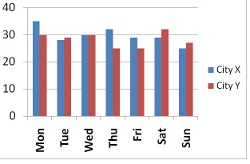Arithmetic
Arithmetic Progression
Associative Property
Averages
Brackets
Closure Property
Commutative Property
Conversion of Measurement Units
Cube Root
Decimal
Divisibility Principles
Equality
Exponents
Factors
Fractions
Fundamental Operations
H.C.F / G.C.D
Integers
L.C.M
Multiples
Multiplicative Identity
Multiplicative Inverse
Numbers
Percentages
Profit and Loss
Ratio and Proportion
Simple Interest
Square Root
Unitary Method
Algebra
Cartesian System
Order Relation
Polynomials
Probability
Standard Identities & their applications
Transpose
Geometry
Basic Geometrical Terms
Circle
Curves
Angles
Define Line, Line Segment and Rays
Non-Collinear Points
Parallelogram
Rectangle
Rhombus
Square
Three dimensional object
Trapezium
Triangle
Trigonometry
Trigonometry Ratios
Data-Handling
Arithmetic Mean
Frequency Distribution Table
Graphs
Median
Mode
Range

Solved Problems
Home >> Graphs >> Double Bar Graph >>

## Double Bar Graph

 Pictograph Bar Graph Double Bar Graph Histogram Pie Graph Line Graph Difference between Bar Graph and Histogram

We hope that you are familiar with Bar Graph and if not, this topic is already here Bar Grap

A single or simple bar graph usually represents value to a single set of data. But when we need to represent and compare two sets of data, we need Double Bar Graphs.

Following example further illustrates Double Bar Graphs.
Example - Temperature of two cities X & Y for entire week is represented in the following tabular form.

 Temperature Mon Tue Wed Thu Fri Sat Sun City X 27 28 30 31 29 31 27 City Y 30 29 30 29 28 32 28

Draw a double bar graph and answer the following questions:<

1) On which day the temperature of both cities is same ?

2) On which day there is maximum difference in temperature of both cities ?

3) On which day there is least difference in temperature of both cities ?

4) Hottest and coldest days(s) for both cities ?

Answer - Below is the required Double Bar Graphs representing temperature of City X and City Y from Monday to Sunday:## Below are answers to questions:

Question 1 - On which day the temperature of both cities is same ?
Answer - The temperature of city X and Y is same on Wednesday i.e 30 degrees

Question 2 - On which day there is maximum difference in temperature of both cities ?
Answer - Maximum difference in temperature of both the cities is on Thursday i.e. 7 degrees difference

Question 3 - On which day there is least difference in temperature of both cities ?
Answer - Least difference in temperature of both the cities is on Tuesday i.e. 1 degree difference only.

Question 4 - Hottest and coldest days(s) for both cities ?